# Introduction to EgoCor

library(EgoCor)

This is an introduction to the package EgoCor. EgoCor offers a user friendly interface displaying by using one function a range of graphics and tables of parameters to facilitate the decision making about which exponential parameters fit either raw data or residuals best. This function is based on the functions of the R package gstat. A further function providing the measure of uncertainty proposed by Dyck and Sauzet (2022) has been implemented in the package. With the R package EgoCor modelling the spatial correlation structure of health outcome with a measure of uncertainly is made available to non specialists.

## Statistical background

Please find more detailed information about the used statistical methods in Dyck and Sauzet (2022).

## Data

The simulated dataset birth is provided with the package EgoCor. The dataset is based on the spatial distribution of real birthweight data. It contains eight variables for 903 births:

• x: x-coordinate in meters for a fictive Cartesian coordinate system;
• y: y-coordinate in meters;
• birthweight: birthweight in gram;
• primiparous : first pregnancy (1) or subsequent pregnancy (0);
• datediff: number of days to due date;
• bmi: BMI of the mother at first medical appointment;
• weight: weight of the mother at first medical appointment;
• inc: income quintile (0, 1, 2, 3, 4).
head(birth)
#>            x           y birthweight primiparous datediff bmi weight inc
#> 1  2760.9530 -2246.30033    3249.693           1       14  23     76   4
#> 2  -747.2682   -16.16918    2429.693           1       22  18     54   4
#> 3 -1609.2821 -3590.09266    3279.693           1       -7  20     59   3
#> 4  8935.2757 -1157.91136    3479.693           1       -1  32    110   3
#> 5 -2258.1243  3226.65995    3569.693           1       -6  23     71   4
#> 6 -6015.6184 -6122.37746    3689.693           0        7  24     75   2

## Functions

We use this dataset to illustrate the following functions:

1. coords.plot(): for graphical description of locations;
2. distance.info(): for descriptive information about distances between observations;
3. vario.mod(): to fit exponential models to semi-variograms with graphical presentation;
4. vario.reg.prep(): to model the spatial correlation structure of residuals of a regression model;
5. par.uncertainty(): to obtain bootstrap standard errors for the parameters of the exponential semi-variogram model.

While detailed information can be retrieved with help(function.name), a presentation and demonstration of all functions is provided in the following sections.

### 1. coords.plot()

The first three columns of the data frame or matrix should be ordered the following way: 1st column: x-coordinate in meters for a Cartesian coordinate system; 2nd column: y-coordinate in meters for a Cartesian coordinate system; 3rd column: outcome of interest. Other columns will be ignored.

The function coords.plot() provides a simple visualization of the locations on a two dimensional map and indicates whether the outcome is observed (by a black circle) or missing (by a red x) at a specific location. The purpose of this function is to look at the spatial distribution of observations and if there might be a spatial pattern in the distribution of missing values in the outcome of interest or in covariates.

coords.plot(birth)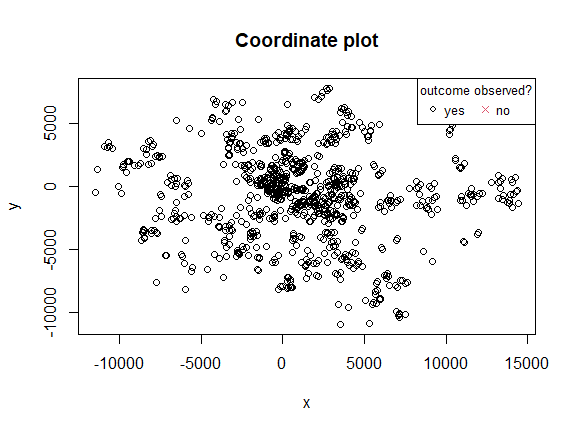### 2. distance.info()

This function provides further information about the distribution of pairwise Euclidean distances. It displays the following descriptive statistics:

• a histogram of Euclidean distances;
• minimum, 1st quartile, median, mean, 3rd quartile and maximum.
distance.info(birth)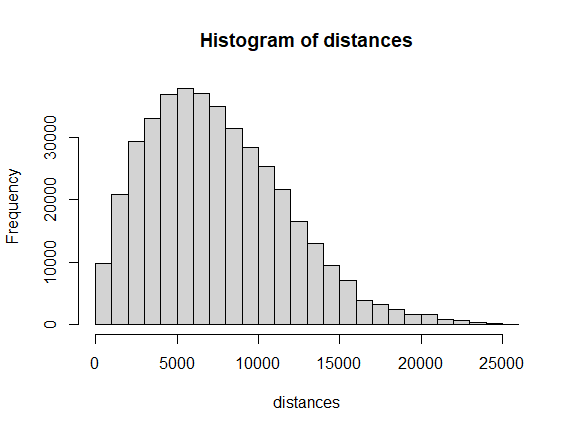#>
#>  Summary of distance set:
#>    Min. 1st Qu.  Median    Mean 3rd Qu.    Max.
#>       0    4244    6970    7506   10221   25963

From all the 815 409 pairwise distances, 30 570 are of less than 2 000 meters and will be used for modelling of the local spatial correlation structure.

### 3. vario.mod()

This function enables the simultaneous output of multiple exponential semi-variogram models fitted for a range of maximal distances and a number of bins. Thereby, the focus lies on the ability of the function to provide multiple estimation results depending on various specifications for the meta parameters max.dist and nbins.

When in the default setting shinyresults = TRUE an interactive shiny application is opened automatically that displays the results. For the purpose of this vignette though we will set shinyresults = FALSE and windowplots = TRUE.

The chosen maximal distance value specifies the subset of data pairs that are actually used within the semi-variogram estimation. Only data pairs with an Euclidean distance ≤ max.dist are taken into account.

For a first exploration, it might be useful to try a range of maximal distances to locate where the range might be situated:

mod = vario.mod(birth, max.dist = c(1000,800,600), shinyresults = FALSE, windowplots = TRUE)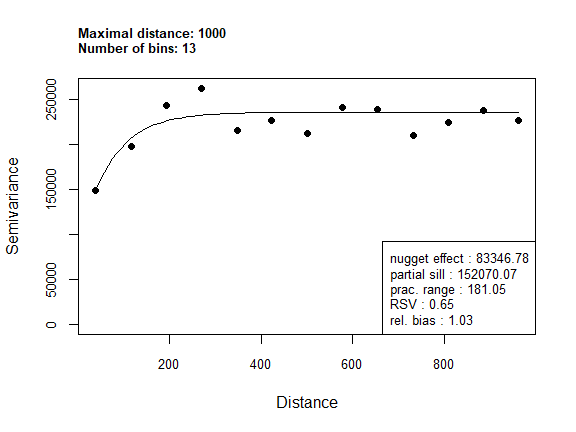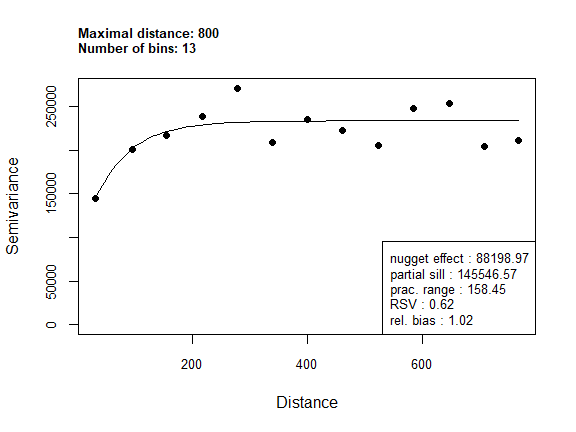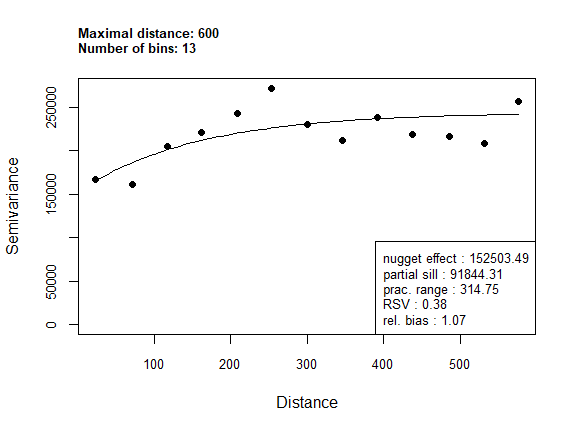#>
#> Parameter estimates:
#>   max.dist nbins nbins.used    nugget partial.sill     shape prac.range
#> 1     1000    13         13  83346.78    152070.07  70.75734   181.0479
#> 2      800    13         13  88198.97    145546.57  62.82642   158.4479
#> 3      600    13         13 152503.49     91844.31 156.03186   314.7528
#>         RSV rel.bias
#> 1 0.6459608 1.028116
#> 2 0.6226710 1.020817
#> 3 0.3758753 1.067119
#>
#> Parameter Legend:
#> Each row contains the estimated parameters of the exponential semi-variogram model with the stated maximal distance.
#>    - Index = model number,
#>    - max.dist = maximal distance considered in the empirical semi-variogram estimation,
#>    - nbins = number of bins specified for the empirical semi-variogram estimation,
#>    - nbins.used = number of bins used for the empirical semi-variogram estimation (can differ from nbins in case of colocatted data points),
#>    - nugget = the estimated nugget effect,
#>    - partial.sill = the estimated partial sill,
#>    - shape = the estimated shape parameter,
#>    - prac.range = the practical range of the exponential semi-variogram model,
#>    - RSV = the relative structured variability,
#>    - rel.bias = the relative bias between sample variance and estimated variance according to the model.
#> 

You can also get the estimated parameters later by

mod$infotable #> max.dist nbins nbins.used nugget partial.sill shape prac.range #> 1 1000 13 13 83346.78 152070.07 70.75734 181.0479 #> 2 800 13 13 88198.97 145546.57 62.82642 158.4479 #> 3 600 13 13 152503.49 91844.31 156.03186 314.7528 #> RSV rel.bias #> 1 0.6459608 1.028116 #> 2 0.6226710 1.020817 #> 3 0.3758753 1.067119 The maximum distance of 800m seems to provide a good fit for this dataset. We can now refine the analysis by varying the number of bins. The nbins parameter specifies the number of lags of the empirical semi-variogram to be estimated. A high number of lags might lead to small within-lag-sample-size and thus to an unstable estimate. Simultaneously, a too small number of lags might lead to a model, that does not detect a spatial correlation structure at all. mod_2 = vario.mod(birth, max.dist = 800, nbins = c(11,12,13), shinyresults = FALSE, windowplots = TRUE)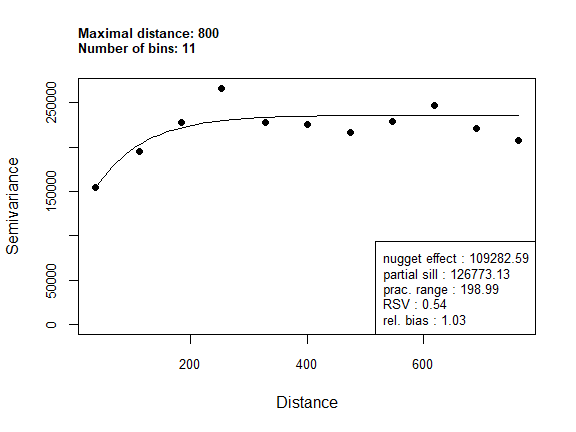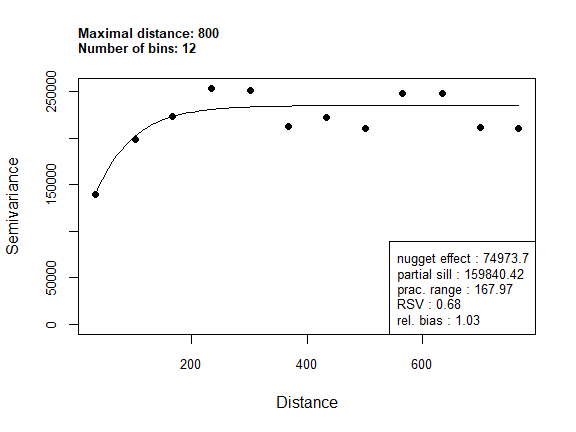#> #> Parameter estimates: #> max.dist nbins nbins.used nugget partial.sill shape prac.range #> 1 800 11 11 109282.59 126773.1 83.81859 198.9907 #> 2 800 12 12 74973.70 159840.4 64.32888 167.9700 #> 3 800 13 13 88198.97 145546.6 62.82642 158.4479 #> RSV rel.bias #> 1 0.5370475 1.030906 #> 2 0.6807104 1.025483 #> 3 0.6226710 1.020817 #> #> Parameter Legend: #> Each row contains the estimated parameters of the exponential semi-variogram model with the stated maximal distance. #> - Index = model number, #> - max.dist = maximal distance considered in the empirical semi-variogram estimation, #> - nbins = number of bins specified for the empirical semi-variogram estimation, #> - nbins.used = number of bins used for the empirical semi-variogram estimation (can differ from nbins in case of colocatted data points), #> - nugget = the estimated nugget effect, #> - partial.sill = the estimated partial sill, #> - shape = the estimated shape parameter, #> - prac.range = the practical range of the exponential semi-variogram model, #> - RSV = the relative structured variability, #> - rel.bias = the relative bias between sample variance and estimated variance according to the model. #>  ### 4. vario.reg.prep() To use vario.mod() to model the spatial correlation structure of residuals, the studentized residuals from a (hierachical) linear regression model can be extracted by vario.reg.prep(). We want to see if adjusting for some predictors of birthweight explain some or all of the spatial correlation structure seen: res <- lm(formula = birthweight ~ datediff + primiparous + bmi, data = birth) v.prep = vario.reg.prep(res, data = birth) models<-vario.mod(v.prep, max.dist = c(800,600), shinyresults = FALSE, windowplots = TRUE)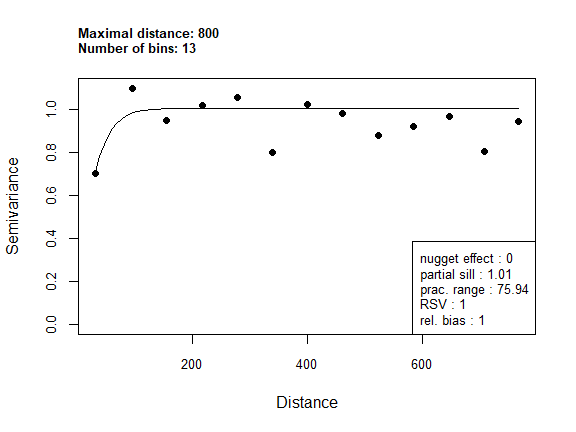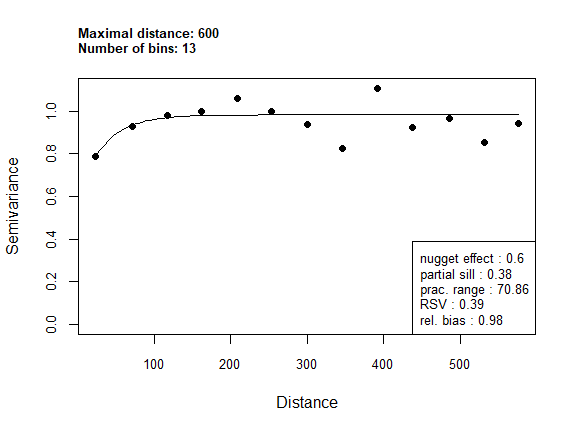#> #> Parameter estimates: #> max.dist nbins nbins.used nugget partial.sill shape prac.range #> 1 800 13 13 0.0000000 1.0055184 25.34775 75.93508 #> 2 600 13 13 0.5998209 0.3824271 34.52489 70.85979 #> RSV rel.bias #> 1 1.0000000 1.0020958 #> 2 0.3893386 0.9789046 #> #> Parameter Legend: #> Each row contains the estimated parameters of the exponential semi-variogram model with the stated maximal distance. #> - Index = model number, #> - max.dist = maximal distance considered in the empirical semi-variogram estimation, #> - nbins = number of bins specified for the empirical semi-variogram estimation, #> - nbins.used = number of bins used for the empirical semi-variogram estimation (can differ from nbins in case of colocatted data points), #> - nugget = the estimated nugget effect, #> - partial.sill = the estimated partial sill, #> - shape = the estimated shape parameter, #> - prac.range = the practical range of the exponential semi-variogram model, #> - RSV = the relative structured variability, #> - rel.bias = the relative bias between sample variance and estimated variance according to the model. #>  The results point towards a reduced spatial correlation structure with a maximal distance reduced to half the one obtained before adjustment and much less regularity in the empirical semi-variogram. ### 5. par.uncertainty() This function provides the filtered bootstrap standard errors for all three exponential model parameters. As the bootstrap can take some time, it is not called by the function vario.mod() directly so that the bootstrap is not executed in all models estimated by par.uncertainty(). This is left to the choice of the user by selecting the model number in the option mod.nr, thus saving execution time. The main output of this function is a table with parameter estimates and standard errors for all three parameters of the estimated model chosen by specifying mod.nr. The user can either provide a model estimated by vario.mod() and a model number that specifies which one to use or provide the parameter estimates, the data, the maximum distance and number of bins seperately. The first option is more convenient, therefore we chose it here. The second option enables standard error estimation for exponential semi-variogram models for which no result of class "vario.mod.output" is available. It is recommended to use more bootstrap samples than done here. unc = par.uncertainty(mod_2, mod.nr = 2, B = 100) #> Two approaches regarding the input arguments: #> 1. Provide the arguments #> - vario.mod.output (output object from vario.mod function), #> - mod.nr (number of the model in the vario.mod.output$infotable).
#>  2. Provide the arguments
#>     - par.est (vector with estimated nugget, partial sill and shape parameters),
#>     - data (used to estimate the semi-variogram model parameters),
#>     - max.dist (semi-variogram parameter, numeric of length 1),
#>     - nbins (semi-variogram parameter, numeric of length 1).
#>  In both cases a threshold factor can be set. If not specified a default value of 1.2 is used.
#>
#>
#>  Bootstrap started.
#>  This can take a few minutes depending on the number
#>  of bootstrap samples B to be generated.
#>
#>
#>  Call:  par.uncertainty(vario.mod.output = mod_2 , mod.nr = 2 , par.est = NULL , data= NULL , max.dist= NULL , nbins = NULL , B = 100 , threshold.factor = 1.2 )
#>
#>                   Estimate  Std. Error
#> nugget effect  74973.69865  90468.9381
#> partial sill  159840.42141 146593.1594
#> shape             64.32888    116.9989
Dyck, Julia, and Odile Sauzet. 2022. “Parameter Uncertainty Estimation for Exponential Semivariogram Models: Two Generalized Bootstrap Methods with Check- and Quantile-Based Filtering.” Preprint. https://doi.org/10.48550/arXiv.2202.05752.